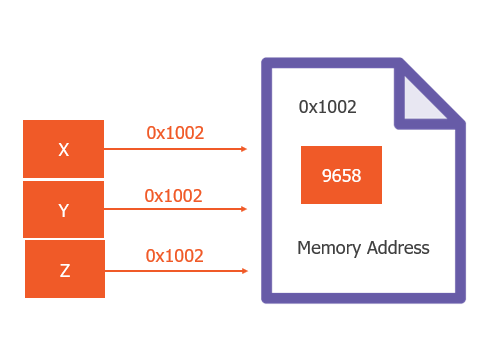# Python Memory Management

Python Jun 26, 2019

In this Article, we will see how memory is assigned to variables in python

By the end of this lesson, you will be able to answer some of the frequently

1.) how does python assigns memory to variables

2.) what is a reference counting

#### Memory

we can think of memory as a series of small chunks in which each piece has a
fixed size and a unique memory address which depends on 64 bit or 32-bit
operating system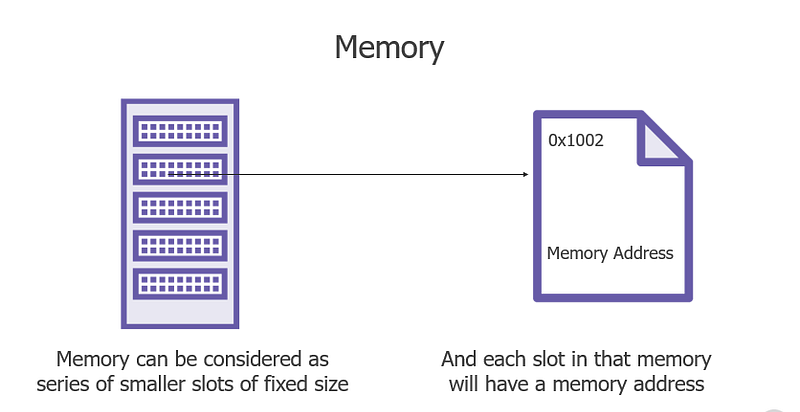Now when we create a variable in python lets say x and assign value 9658 to it

``````X=9658
``````

then internally what it will do is it stores the value that is 9658 in one of
the memory slot (also known as heap memory), and the identifier that is x is
nothing more than the reference to that memory address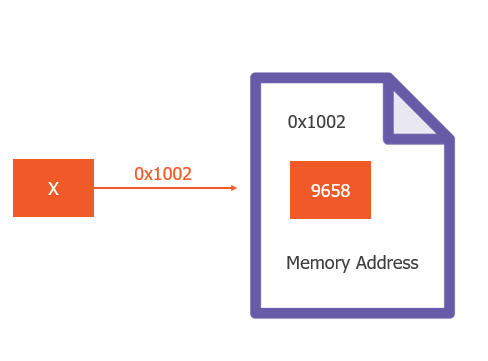so the point is the X is not equal to 9658 it is a reference to the memory

Now as I told you the memory slot has a limited size

so what if we have an object that is greater in size than the slot let say

we have a list of 2000 elements

``````lst = [i for i in range(0,2000)]
``````

so, in this case, the memory the data will flow from one slot to another if the
size of data is greater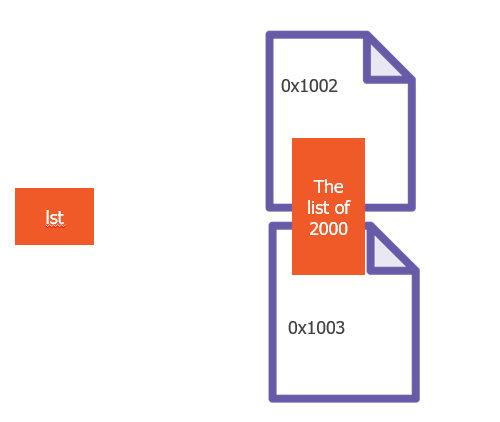Note: it does not matter how many slots are used to store data the identifier
(lst in our case) will always point to the address of first memory slot where
the data storage started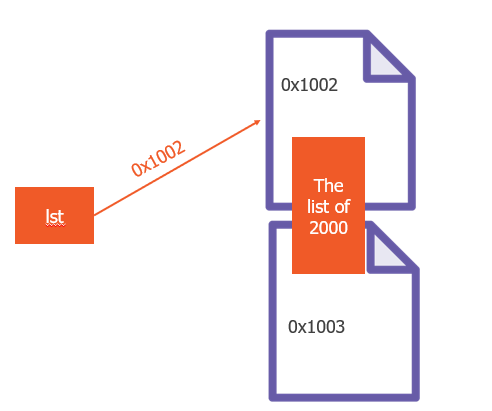now if X is only a pointer, then we can assign this pointer to another
identifier also right

``````X=9658
Y=X
``````

the above code will not generate a new variable Y with value 9658

rather we are asking Y to go ahead and point to the same memory address where X
is pointing to.

Now, to prove it we have one function in python that is id() which returns the
memory address of the object in base 10, and we can convert that base 10 to

``````X=9658
pint(hex(id(X)))

Y=X
pint(hex(id(Y)))
``````

so if you will run this snippet, we will get the same memory address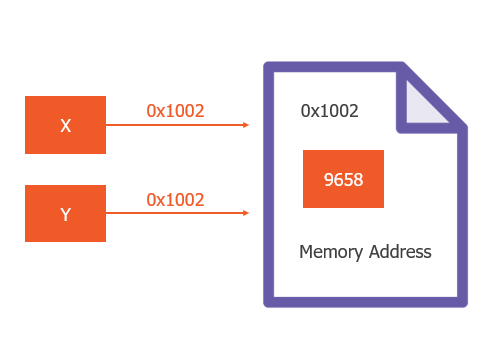#### Reference counting

Reference counting is the count of identifiers pointing to a single memory

the reference count of a memory address where x is pointing to is 2 in our case
if we assign one more variable then it will become 3

``````X=9658
Y=X
Z=Y
``````